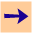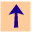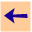6.3 Depth Bound

## 6.3 Depth Bound

The search strategy used by CILOG is a depth-bounded depth-first search. The depth bound is set using the command:
```cilog: bound n.
```
where n is a positive integer. n is a bound on the depth of any proof tree. The default value is 30.

When a goal is asked and no more answers can be found, if the depth-bound was not reached, the system reports there are no more answers. If the depth-bound was reached, there still may be some answers, although it is more likely that there is a bug in the knowledge base where some recursions do not terminate. CILOG allows the user to interactively explore the places where the depth bound was reached.

When no more answers can be found and the search was cut off due to hitting the depth-bound, the user is informed of this, as is given the option of one of:

i.
where i is an integer bigger than the current depth-bound. This lets the user explore larger search trees. Repeatedly increasing the depth-bound lets the user simulate an iterative deepening search.
where.
to let the user explore the place where the depth-bound was reached.
ok.
to go back to the answer prompt.
help.
for a list of the available commands.

When the user given the where command, the proof is retried, and it halts at a point where the depth-bound was reached, and shows the user the current subgoal, g. The user can give one of the following commands:

fail.
to fail g, and explore other places where the depth-bound was reached.
succeed.
to say that g should succeed.
proceed.
to fail g and not explore any more subgoals where the depth-bound was reached.
why.
to let the user explore why g was called (see Section 6.4).
ok.
to go back to the answer prompt.
help.
for a list of the available commands.6.3 Depth Bound